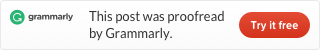Categories

# LeetCode 164 Maximum Gap [sort] java

Given an unsorted array, find the maximum difference between the successive elements in its sorted form.

Return 0 if the array contains less than 2 elements.

Example 1:

```Input: [3,6,9,1]
Output: 3
Explanation: The sorted form of the array is [1,3,6,9], either
(3,6) or (6,9) has the maximum difference 3.```

Example 2:

```Input: 
Output: 0
Explanation: The array contains less than 2 elements, therefore return 0.```

This problem is easy. First, we sort, then loop the data, find the maximum difference.